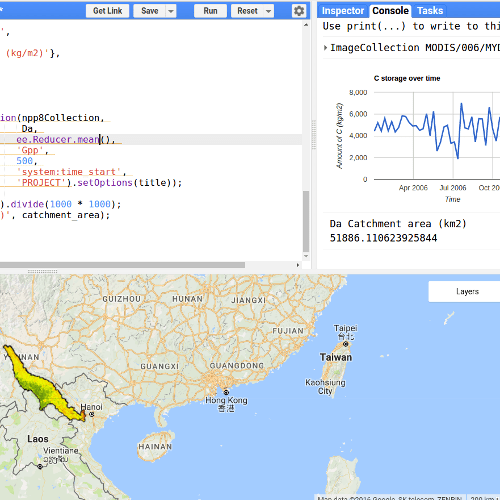# Calculate Carbon storage in a watershed

How much carbon do all these plants and trees store?

This tutorial describes how to build a script that calculates the total carbon storage in a watershed using the Google Earth Engine.

In this example we use the Net Primary production (NPP) and Gross Primary Production (GPP) products from the MODIS aqua satellite. NPP is available on the yearly timescale, the GPP for every 8 days. We use the GPP to calculate NPP for every 8 days using the fractional yearly contribution. A good explanation of GPP and NPP can be found here.

1. Import the “MYD17A2H.006: Gross Primary Productivity 8-Day L4 Global 500 m” and “MYD17A3H.006: Net Primary Production Yearly L4 Global 500 m”.2. Import the Da basin into the GEE.

```// import the sub basins as a fusion table
var Da = ee.FeatureCollection('ft:15ytieQkq3UH8DPJPFmB_YnbvhSEhOQLtLIXa6kxA').geometry();

// Set center of map
Map.centerObject(Da, 6);

// show the layer
```

3. Filter the image collection based on location and time.

```// set start and end date
var startdate = ee.Date.fromYMD(2006,1,1);
var enddate = ee.Date.fromYMD(2006,12,1);

// filter npp
var nppCollection = npp.filterDate(startdate, enddate)
.filterBounds(Da)
.select(&quot;Npp&quot;);
// filter gpp
var gppCollection = gpp.filterDate(startdate, enddate)
.filterBounds(Da)
.select(&quot;Gpp&quot;);

```

4. Create a function to calculate the 8-daily npp using the gpp

```var myNpp = function(myimg){
// get date
var d = ee.Date(myimg.get('system:time_start'))
// get year
var y = d.get('year').toInt();

// filter for year for GPP and NPP
var GPPy = ee.Image(gppCollection.filter(ee.Filter.calendarRange(y, y, 'year')).sum());
var NPPy = ee.Image(nppCollection.filter(ee.Filter.calendarRange(y, y, 'year')).mean());
var npp8 = myimg.expression('(GGP8 / GPPy) * NPPy',
{
GGP8: myimg,
GPPy: GPPy,
NPPy: NPPy
});

// multiply with scale factor
npp8 = npp8.multiply(??);

return npp8.copyProperties(myimg,['system:time_start'])
}

```

5. Run the function you just create for all images in the gppCollection

```var npp8Collection = ee.ImageCollection(gppCollection.map(myNpp));
```

6. Setup some vizualizaton paramaters

```// create vizualtion settings
var npp_viz = {min:0.0, max:2, palette:&quot;ff0000,f0ff00,004717&quot;};
```

7. Add the image to the Canvas:

```// add the image
```

8. Plot a chart for the area of interest

```
// Predefine the chart titles.
var title = {
title: 'C storage over time',
hAxis: {title: 'Time'},
vAxis: {title: 'Amount of C (kg/m2)'},
};

// print the chart
print(Chart.image.seriesByRegion(npp8Collection,
Da,
ee.Reducer.mean(),
'Gpp',
500,
'system:time_start',
'PROJECT').setOptions(title));
```

9. Print the total area of the basin

```var catchment_area = Da.area().divide(1000 * 1000);
print('Da Catchment area (km2)', catchment_area);
```

See an example here

1.Pun Wath says:
1.thisearthsite says: